#### Symbolab – Math solver v5.3.2 (Pro) APK

Calculators with steps: equations, integrals, derivatives, limits, trig and more
##### Details
Title: Symbolab - Math solver
Genre: Education
Developer: Symbolab
##### Description

Your private math tutor, solves any math problem with steps! Equations, integrals, derivatives, limits and much more.
(Steps require an in-app subscription)

Symbolab Math Solver app is composed of over one hundred of Symbolab's most powerful calculators:
Equation Calculator
Integral Calculator
Derivative Calculator
Limit calculator
Inequality Calculator
Trigonometry Calculator
Matrix Calculator
Functions Calculator
Series Calculator
ODE Calculator
Laplace Transform Calculator
Download the app to experience the full set of Symbolab calculators.

Symbolab Math Solver solves any math problem including Pre- Algebra, Algebra, Pre-Calculus, Calculus, Trigonometry, Functions, Matrix, Vectors, Geometry and Statistics.

##### Screenshots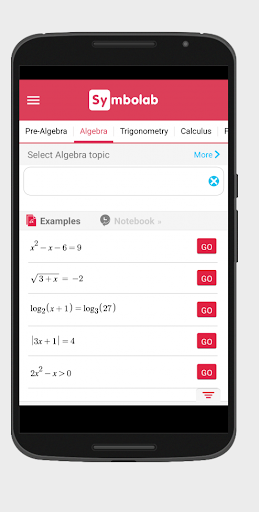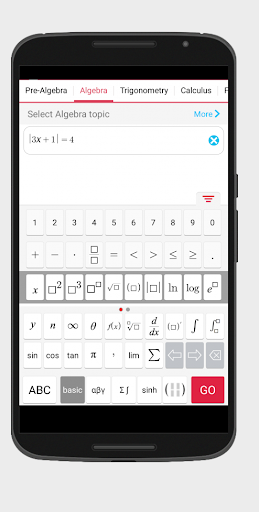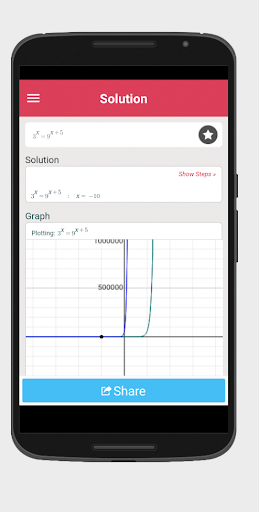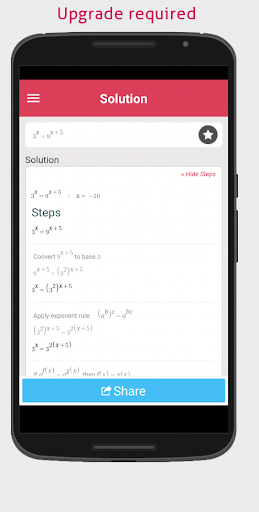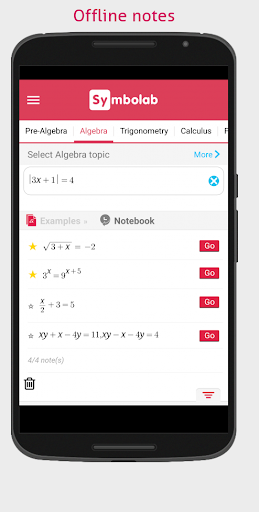##### Changelog

– Arabic support
– Enhancements and bugfixes